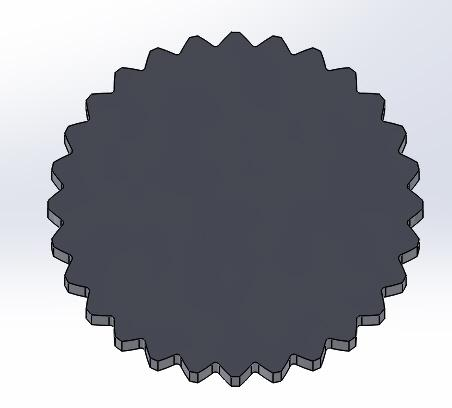# Involute profile in splines (2)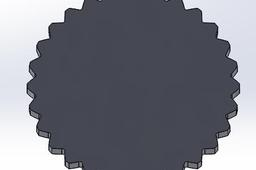This is a continuation of the previous tutorial Involute profile in splines (1)

1. ### Step 1: Involute function

Let's define an involute function.

inv(α)=tan(α)−α=φ  (All angles for the involute function must always be given in radians)

With the involute function, many geometric spline parameters can be calculated. For example, the involute function can be used to determine the tooth thickness "s" on an arbitrary diameter "d" of a spline.

Pressure angle "α0" (defined in https://grabcad.com/tutorials/involute-profile-in-splines-1)

"s0" denotes the tooth thickness on the reference pitch circle

"d0" denotes the diameter of the reference pitch circle. (r0= d0/2)

See figure below. (1)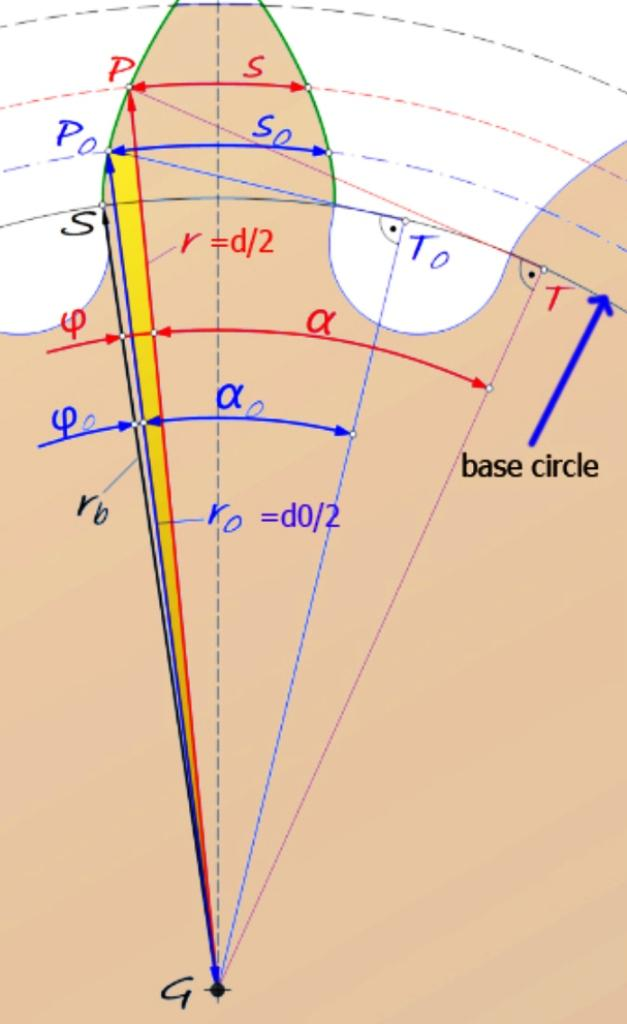2. ### Step 2: Involute profile parameters

Pitch circle diameter can be found in tables of the different standards.

The tooth thickness at the considered distance "r" to the center of the base circle G is denoted by "s".

s=d*((s0/d0)+inv(α0)–inv(α))

α=arccos((d0/d)⋅cos(α0))

The diameter of the circle at which involute begins and the diameter of which involutes ends can be found in tables of the different standards.

Take as an example an ANSI 92.1 external spline with a pressure angle of 45 deg, spline pitch 24/48, and 28 teeth. See figures below (in red involute profile) (2 y3)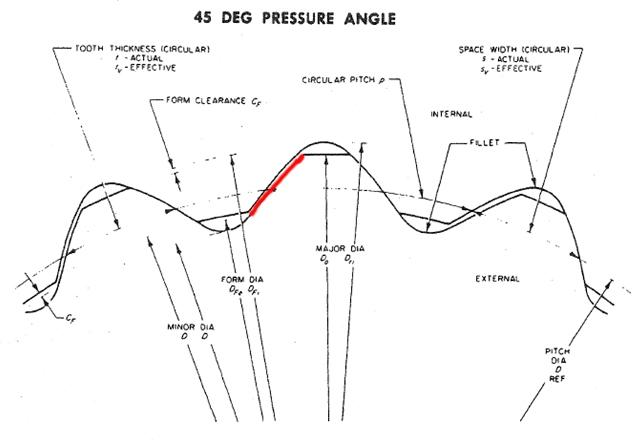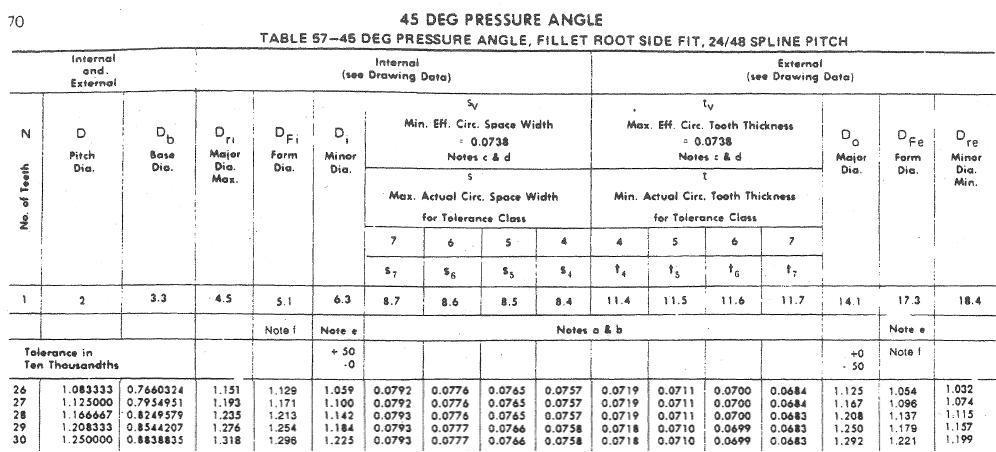3. ### Step 3: External involute

The main values of the parameters can be seen below (4). In yellow the diameters of the limits circles of involute profile.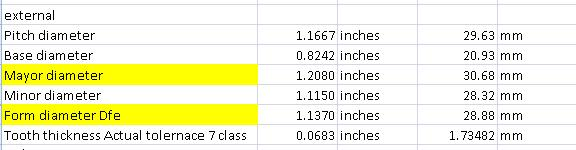4. ### Step 4: Calculation of the tooth thickness

Let's take the minor diameter, pitch diameter, and mayor diameter.

Depending on the required precision, the number of points used to trace the involute profile.

(pitch diameter - Minor diameter)/4 =0.33 mm

(mayor diameter - pitch diameter)/3 = 0.35 mm

α=arccos((d0/d)⋅cos(α0))

inv(α)=tan(α)−α

s=d*((s0/d0)+inv(α0)–inv(α))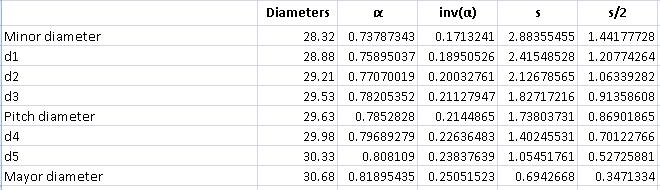5. ### Step 5: Draw of arcs

Let's draw the arcs with the predetermined diameters and lengths.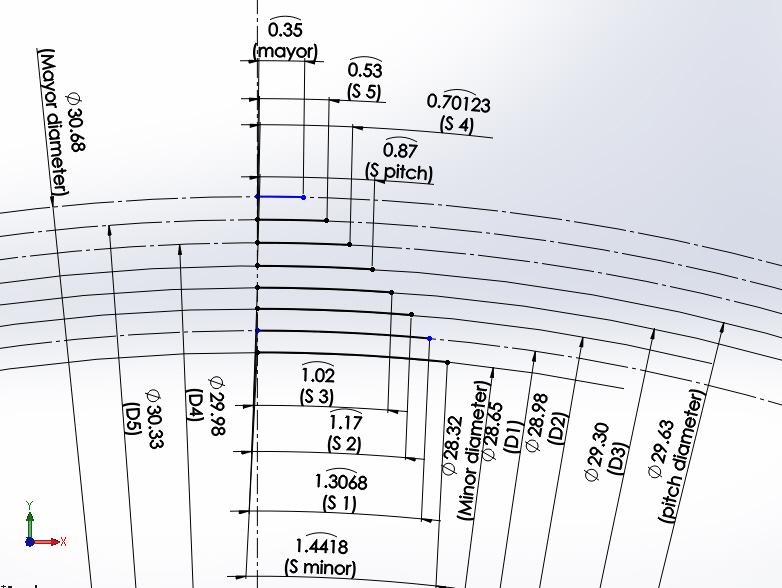6. ### Step 6: Curve tracing

Let's connect the points. ( I used a spline in Solidworks)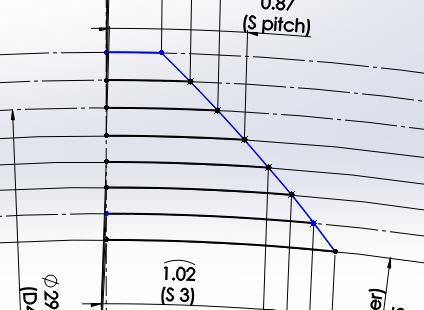7. ### Step 7:

The involute profile does not reach the smallest diameter. We must cut (trim) this part of the curve.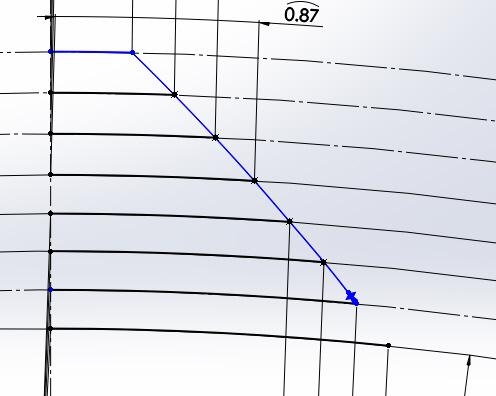8. ### Step 8: Tooth tracing

The fillet between the involute profile and the Minor circle depends on the tool used to machining the tooth. ( exceeds this tutorial). The fillet must be tangent to the involute profile and the minor circle

In our case, the spline has 28 teeth, then 360 deg/28 =12.857 deg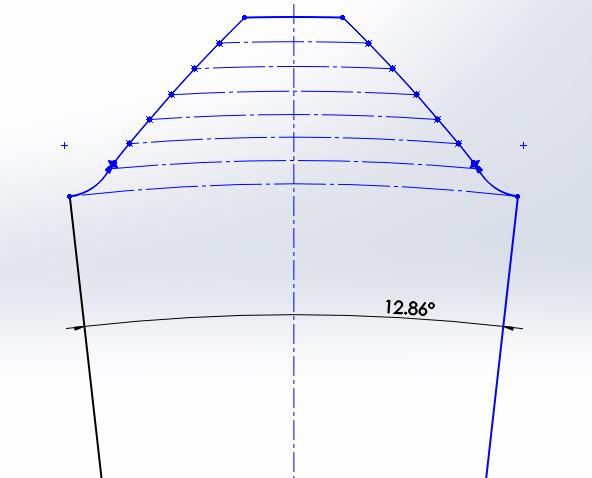9. ### Step 9: Extrude tooth.

Extrude tooth at the desired thickness.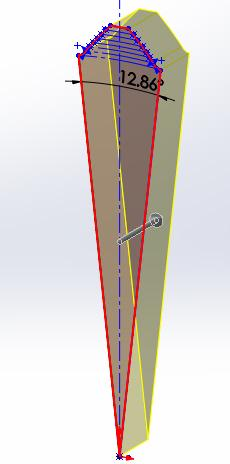10. ### Step 10: Array of N teeth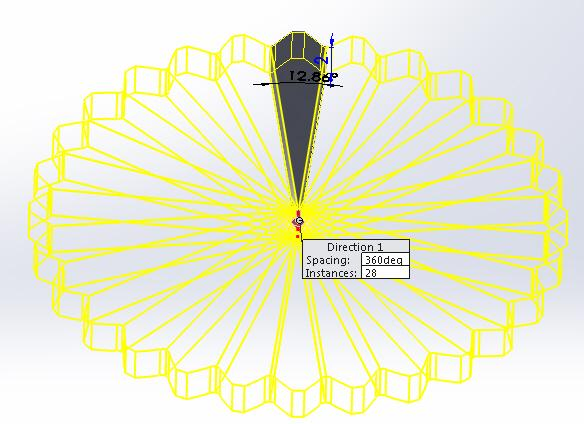11. ### Step 11: Final